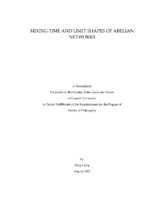## MIXING TIME AND LIMIT SHAPES OF ABELIAN NETWORKS##### Abstract
Activated Random Walk (ARW) is an interacting particle system on the $d$-dimensional lattice $\Z^d$ with two types of particles: Active particles perform random walk and fall asleep at a fixed rate $\lambda$, and sleeping particles wake up if an active particle encounters them. On a finite subset $V \subset \Z^d$ it defines a Markov chain on $\{0,1\}^V$. We prove that when $V$ is a Euclidean ball intersected with $\Z^d$, the mixing time of the ARW Markov chain is at most $1+o(1)$ times the volume of the ball. The proof uses an exact sampling algorithm for the stationary distribution, a coupling with internal DLA, and an upper bound on the time when internal DLA fills the entire ball. These results are joint work with Lionel Levine. The stationary state of the ARW Markov chain on an interval of length n is conjectured to have a limit as n goes to infinity, and the limit is conjectured to have hyperuniformity. As a step toward proving these conjectures, we derive differential equations describing how the stationary state changes with $\lambda$ and n. Spinner Sandpile is a variant of the Abelian Sandpile Model. We prove outer and inner bounds for the stabilized configuration of Spinner Sandpiles on $\mathbb{Z}^d$ starting from a point source of particles at the origin.
111 pages
2022-08
##### Keywords
Abelian Network; Activated Random Walk; Probability; Sandpile
Levine, Lionel
##### Committee Member
Moore, Justin Tatch
Sosoe, Philippe
Mathematics
##### Degree Name
Ph. D., Mathematics
##### Degree Level
Doctor of Philosophy